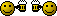# Flow calculations for viscosity

Status
Not open for further replies.

#### Ethan

Can somebody tell me how to calculate variances in flow rates based on differentials in viscosity?

There isn't a simple, arbitrary formula for the issue you describe. Differening kinematic viscosities will lead to differening Reynolds numbers, which in turn requires different methods of calculation, or in the more practical sense, approximation using correlations. http://hyperphysics.phy-astr.gsu.edu/hbase/pfric.html has a rough rundown of some of the very simple formulas as they apply to fluids that exhibit laminar flow characteristics. As you can appreciate, the whole area of fluid mechanics is a very complicated topic, and there are many empirical (involving experimentation, and the development of correlations), and theoretical (using involving some form of expression of the Navier-Stokes equation) to describe fluid characteristics, including flow as it relates to viscosity.

This will get you started on Navier-Stokeshttp://en.wikipedia.org/wiki/Navier_Stokes_Equations Having fun yet?Seriously though, the way that the relationships are determined in the real world is through either wind tunnel/actual fluid flow experimentation (depending on the fluid of interest), or through extremely complex series' of numerically solved partial differential equations (aka the finite element/different method of analysis). [ March 31, 2005, 03:16 AM: Message edited by: pitzel ]

quote:
Originally posted by pitzel: This will get you started on Navier-Stokeshttp://en.wikipedia.org/wiki/Navier_Stokes_Equations Having fun yet?Yep. That should give him a taste of what it takes to become a degreed engineer.boy oh boy... one of my favorite times in school was deriving and solving navier-stokes equations for all sorts of weird situations... Nope, not at all... Im glad that is over and done. Have fun! JMH Delaware CHEG 02 Rowan CHEG 04

With a few reasonable assumptions, the flow of liquids is not as complicated as it may seem. Most liquids compress so little that they may be treated as incompressible in real world calculations. Most of the oil base stocks are close to newtonian fluids, viscosity changing little with shear. A completely formulated motor oil is not quite as close, but still often can be treated as such in the real world. Flow through a pipe is linear with pressure, length, and viscosity. Twice the pressure, or half the viscosity or length, gives twice the flow. The flow varies with the 4'th power of the radius for laminar flow or the 5'th power for turbulent flow. If you lay ahold of enough data, you can calculate the Reynolds number to determine which to use. Either way, the size of the passage way has a enormous effect on flow, about 16 or 32 times more flow for twice as big of a pipe. With the above assumptions, you can understand much of what is going on, and solve many real world problems.

quote:
Originally posted by labman: With the above assumptions, you can understand much of what is going on, and solve many real world problems.
Another way to say that is if you use the right model, you can simplify the calculations substantially. Ethan, here's a link that may get you started: Fluid Flow and Dynamics. Look at slide 10 & 11, Poiseuille's law. [ April 01, 2005, 12:02 AM: Message edited by: 427Z06 ]

Status
Not open for further replies.